## hexx

Hexagonal utilities

### 19 releases(9 breaking)

 0.10.1 Sep 18, 2023 Jul 28, 2023 Mar 26, 2023

#43 in Math

Apache-2.0

3.5MB
3.5K SLoCHexagonal tools lib in rust.

Inspired by this `RedBlobGames` article and Sander Evers work

This lib allows you to:

• Manipulate hexagon coordinates
• Generate hexagonal maps with custom layouts and orientation
• Generate hexagon meshes (planes or columns)

I made the choice to use Axial Coordinates for performance and utility reasons, but the [`Hex`] type has conversion utilities with cubic, doubled and offset coordinates.

See the hexagonal coordinate systems

## Installation

Run `cargo add hexx` in your project or add the following line to your `Cargo.toml`:

• `hexx = "0.10"`

### Cargo features

`hexx` supports serialization and deserialization of most types using serde, through the `serde` feature gate. To enable it add the following line to your `Cargo.toml`:

• `hexx = { version = "0.10", features = ["serde"] }`

By default `Hex` uses rust classic memory layout, if you want to use `hexx` through the FFI or have `Hex` be stored without any memory padding, the `packed` feature will make `Hex` `repr(C)`. To enable this behaviour add the following line to your `Cargo.toml`:

• `hexx = { version = "0.10", features = ["packed"] }`

`hexx` supports Bevy Reflection through the `bevy_reflect` feature. To enable it add the following line to your `Cargo.toml`:

• `hexx = { version = "0.10", features = ["bevy_reflect"] }`

## Features

`hexx` provides the [`Hex`] coordinates with:

• Distances
• Neighbors and directions
• Lines
• Ranges
• Rings
• Edges
• Wedges
• Spirals
• Rotation
• Symmetry
• Vector operations
• Conversions to other coordinate systems:
• Cubic coordinates
• Offset coordinates
• Doubled coordinates
• Hexmod coordinates
• Multiple hex resolution

## Basic usage

`````` use hexx::*;

// Declare points in hexagonal spaces
let point_a = hex(10, -5); // Equivalent of `Hex::new(10, -5)`
let point_b = hex(-8, 15);
// Find distance between them
let dist = point_a.unsigned_distance_to(point_b);
// Compute a line between points
let line: Vec<Hex> = point_a.line_to(point_b).collect();
// Compute a ring from `point_a` containing `point_b`
let ring: Vec<Hex> = point_a.ring(dist).collect();
// Rotate `point_b` around `point_a` by 2 times 60 degrees clockwise
let rotated = point_b.rotate_cw_around(point_a, 2);
// Find the direction between the two points
let dir_a = point_a.main_direction_to(point_b);
let dir_b = point_b.main_direction_to(point_a);
assert!(dir_a == -dir_b);
// Compute a wedge from `point_a` to `point_b`
let wedge = point_a.wedge_to(point_b);
// Get the average value of the wedge
let avg = wedge.average();
``````

## Layout usage

`HexLayout` is the bridge between your world/screen/pixel coordinate system and the hexagonal coordinates system.

`````` use hexx::*;

let layout = HexLayout {
hex_size: Vec2::new(1.0, 1.0),
orientation: HexOrientation::Flat,
..Default::default()
};
// Get the hex coordinate at the world position `world_pos`.
let world_pos = Vec2::new(53.52, 189.28);
let point = layout.world_pos_to_hex(world_pos);
// Get the world position of `point`
let point = hex(123, 45);
let world_pos = layout.hex_to_world_pos(point);
``````

## Wrapping

`HexBounds` defines a bounding hexagon around a center coordinate. It can be used for boundary and interesection checks but also for wrapping coordinates. Coordinate wrapping transform a point outside of the bounds to a point inside. This allows for seamless or repeating wraparound maps.

``````use hexx::*;

let center = hex(23, -45);
let outside_coord = hex(12345, 98765);
assert!(!bounds.is_in_bounds(outside_coord));
let wrapped_coord = bounds.wrap(outside_coord);
assert!(bounds.is_in_bounds(wrapped_coord));
``````

## Resolutions and chunks

[`Hex`] support multi-resolution coordinates. In practice this means that you may convert a coordinate to a different resolution:

• To a lower resolution, meaning retrieving a parent coordinate
• to a higher resolution, meaning retrieving the center child coordinate

Resolutions are abstract, the only useful information is the resolution radius.

For example, if you use a big grid, with a radius of a 100, you might want to split that grid evenly in larger hexagons containing a 10 radius of coordinates and maybe do operations locally inside of these chunks.

So instead of using a big range directly:

``````use hexx::*;

// Our big grid with hundreds of hexagons
``````

You may define a smaller grid you will then divide to a higher resolution

``````use hexx::*;

for chunk in chunks {
// We can retrieve the center of that chunk by increasing the resolution
// And retrieve the other coordinates in the chunk
// We can retrieve the chunk coordinates from any coordinate..
for coord in children {
// .. by reducing the resolution
}
}
``````

An other usage could be to draw an infinite hex grid, with different resolutions displayed, dynamically changing according to user zoom level.

## Usage in Bevy

If you want to generate 3D hexagonal mesh and use it in bevy you may do it this way:

`````` use bevy::{
prelude::Mesh,
render::{mesh::Indices, render_resource::PrimitiveTopology},
};
use hexx::MeshInfo;

pub fn hexagonal_plane(mesh_info: MeshInfo) -> Mesh {
let mut mesh = Mesh::new(PrimitiveTopology::TriangleList);
mesh.insert_attribute(Mesh::ATTRIBUTE_POSITION, mesh_info.vertices);
mesh.insert_attribute(Mesh::ATTRIBUTE_NORMAL, mesh_info.normals);
mesh.insert_attribute(Mesh::ATTRIBUTE_UV_0, mesh_info.uvs);
mesh.set_indices(Some(Indices::U16(mesh_info.indices)));
mesh
}
``````

The `MeshInfo` can be produced from `PlaneMeshBuilder` or `ColumnMeshBuilder`

See the examples for bevy usage

## Examples

`hexx` provides interactive examples showcasing various features:

### Hex grid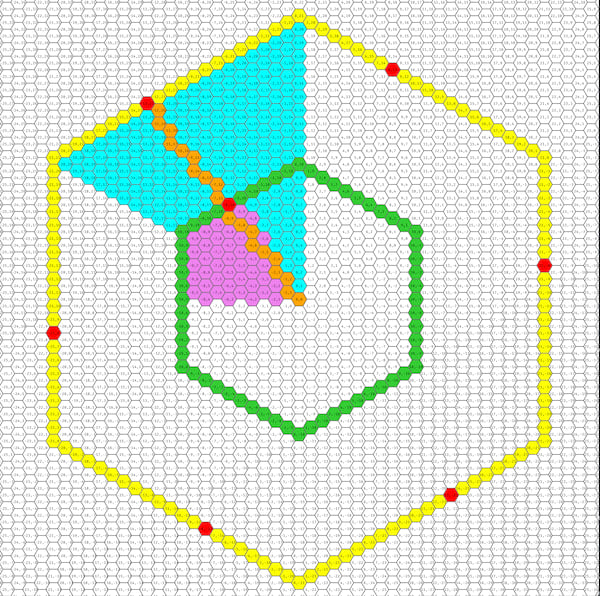`cargo run --example hex_grid`

This example showcases hex ranges, rings, wedges, rotation, and lines

### Scroll Map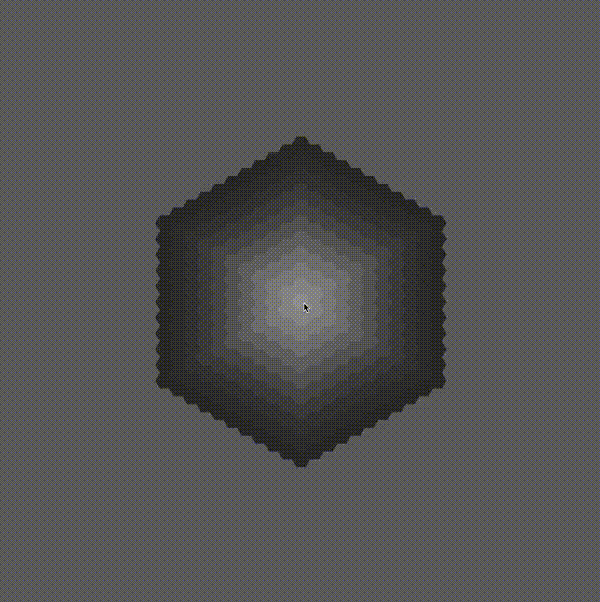`cargo run --example scroll_map`

This example showcases the `HexMap` struct for scrolling maps

### Wrap Map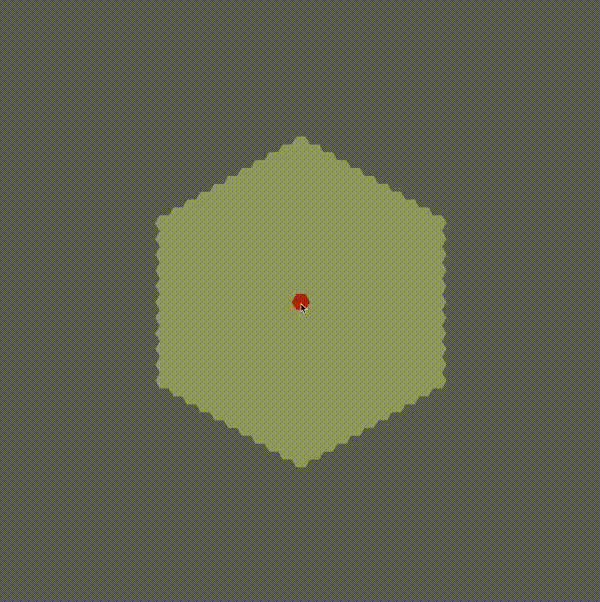`cargo run --example wrap_map`

This example showcases the `HexMap` struct for looping/wrapping map

### A Star pathfinding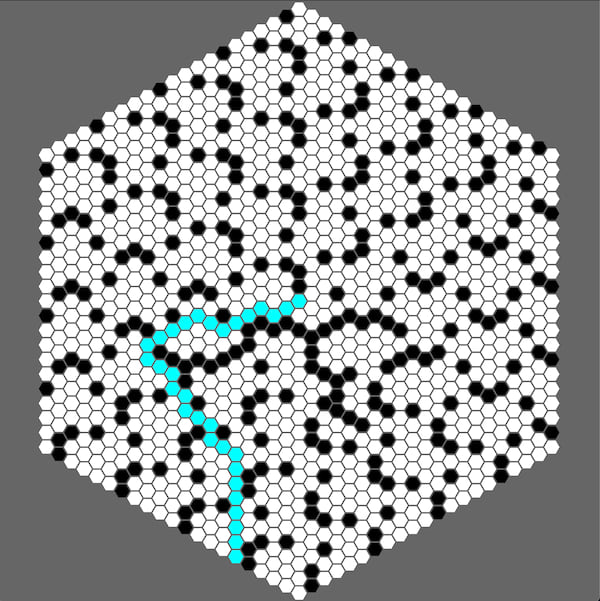`cargo run --example a_star`

This example showcases the A star algorithm, with an interactive pathfinding between the origin and your cursor. Clicking on tile toggles their availability

### Field of view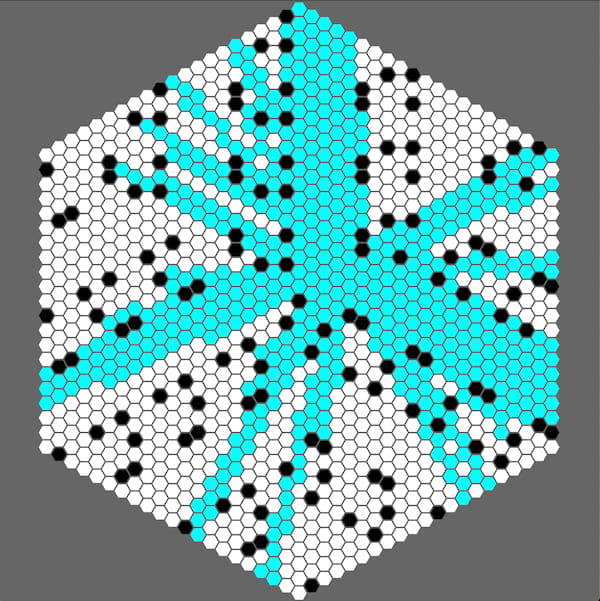`cargo run --example fov`

This example showcases the FOV algorithm, with an interactive range fov around your cursor. Clicking on tile toggles their visibility.

### Field of movement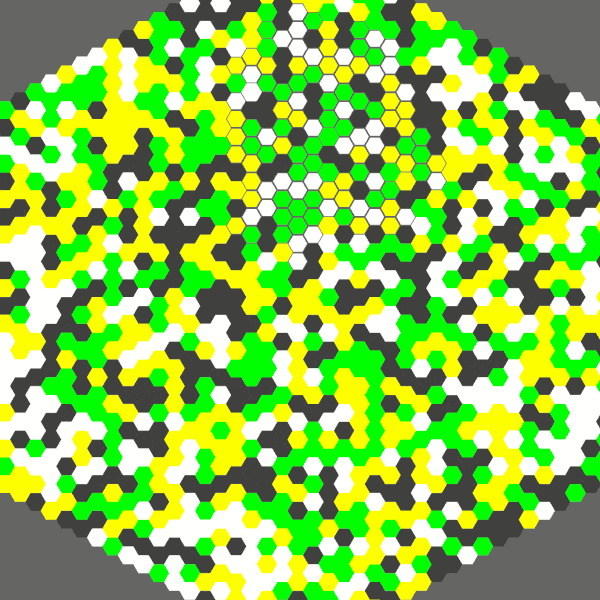`cargo run --example field_of_view`

This example showcases the field of movement algorithm, interactively displaying the accessible range of movement around the cursor.

### 3d columns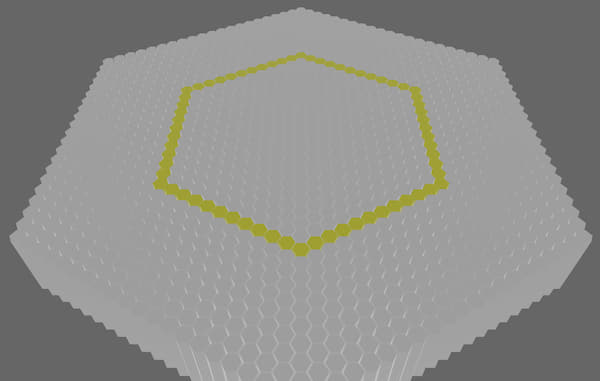`cargo run --example 3d_columns`

This example showcases the 3d hexagon columns procedural generation

### Mesh builder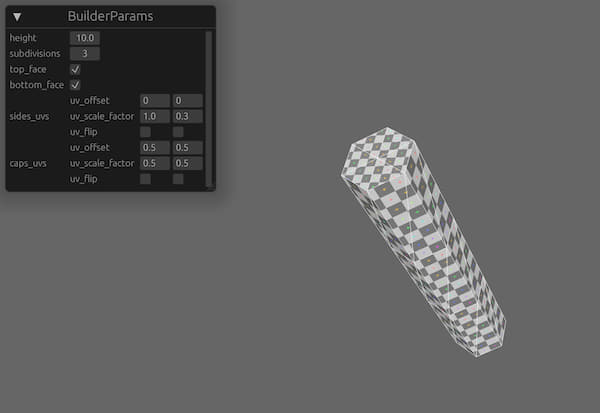`cargo run --example mesh_builder --features bevy_reflect`

This example showcases the hexagon columns procedural generation customization options

### Chunks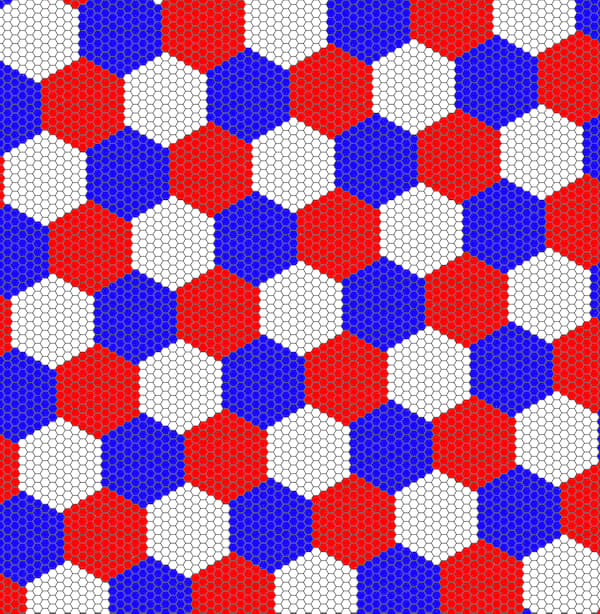`cargo run --example chunks`

This example showcases the hexagon resolution system, allowing to tile coordinates in evenly sized chunks

### Merged Chunks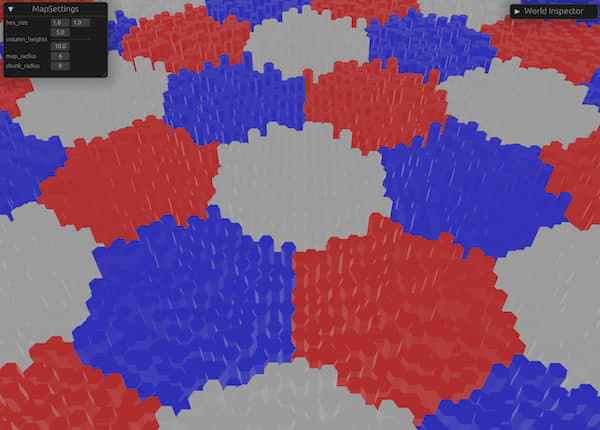`cargo run --example merged_columns --features bevy_reflect`

This example showcases how to build a simple hex chunk system with each chunk being a single mesh

~2.1–10MB
~105K SLoC